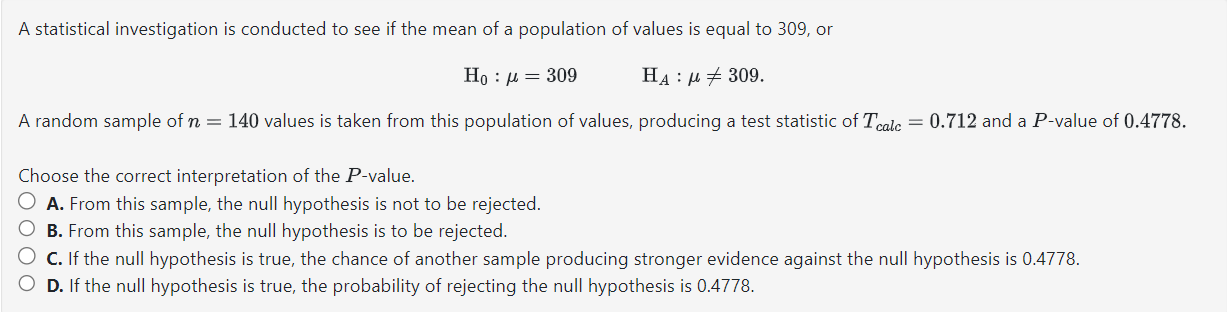Home / Expert Answers / Statistics and Probability / a-statistical-investigation-is-conducted-to-see-if-the-mean-of-a-population-of-values-is-equal-to-pa696

# (Solved): A statistical investigation is conducted to see if the mean of a population of values is equal to ...A statistical investigation is conducted to see if the mean of a population of values is equal to 309 , or A random sample of values is taken from this population of values, producing a test statistic of and a -value of 0.4778 . Choose the correct interpretation of the -value. A. From this sample, the null hypothesis is not to be rejected. B. From this sample, the null hypothesis is to be rejected. C. If the null hypothesis is true, the chance of another sample producing stronger evidence against the null hypothesis is 0.4778 . D. If the null hypothesis is true, the probability of rejecting the null hypothesis is 0.4778 .

We have an Answer from Expert